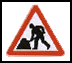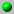Eric Foxley's Music DatabaseStatistics of the tunes

Music database home
The tunes
The PDF pages
[The scores]
[Browse the tunes]
[Find similar tunes]
[The statistics]
[The database]
Storing music
Music analysis

Options in [] I'm working on.

This database stores about 1200 folk melodies, mostly intended for dancing. I have researched the analysis of such tunes, and some of the incidental statistics are included here. These statistics are used in determining the similarity between tunes.

 Note length Notes Pitches Intervals between notes JigsReelsHornpipesWaltzesMorris (a few)Slip jigsPlayford etc. (few)Mick Peat's tunesWe analyse for each category (jigs, reels, etc.) of tune
• the lengths of all the notes, and show the tunes in order of average note length, with histogram percentage counts of the different note lengths. The columns are
• the number of notes
• the average note length
• the percentage of notes less than or equal to a demi-semi-quaver
• the percentage of notes more than this length up to a dotted demi-semi-quaver
• the percentage of notes more than this length up to a semi-quaver
• the percentage of notes more than this length up to a dotted semi-quaver
• ...
• the average length of the last notes of each bar
• the ratio of the average note length to the average last-note-of-the-bar length
• the key of the original
• the title
• the pitches of all note relative to the key the tune is in, and show the percentage of notes at each pitch
• the number of notes
• the average note length
• ....
• the percentage of notes on the fourth of the key (i.e. F w.r.t the key of C)
• the percentage of notes on the root of the key
• the percentage one fifth up
• the percentage one tone up
• ....
• the key of the original
• the title
• the pitch intervals between consecutive notes, and show the percentages of the number of times each interval occurs
• the number of notes
• the average note length
• ....
• the percentage of intervals up one note
• the percentage of unison (or octave) note intervals
• the percentage of intervals down one note
• the percentage of intervals down two notes
• ....
• the key of the original
• the title

Try also the tunes directory.

 Greenwood Clog Foresters Morris Freds Folks King Billy pub singing sessions
Site under recovery from an ancient version, latest update 12-Jan-2021